Practice the AP 9th Class Physical Science Bits with Answers Chapter 1 Motion on a regular basis so that you can attempt exams with utmost confidence.

## AP State Syllabus 9th Class Physical Science Bits 1st Lesson Motion with Answers

Question 1.
A body released from height ‘h’ takes time’t’ to reach the ground. After time t/2, its height from the ground is
A) $$\frac{\mathrm{h}}{2}$$
B) $$\frac{\mathrm{h}}{4}$$
C) $$\frac{3 h}{4}$$
D) $$\frac{\mathrm{h}}{3}$$
C) $$\frac{3 h}{4}$$

Question 2.
Which of the following is correct ?
A) Distance ≥ Displacement
B) $$\frac{\text { Distance }}{\text { Displacement }}$$ ≥ 1
C) Both A & B
D) None
C) Both A & BQuestion 3.
Ratio of the distance travelled in 1 second, 2 seconds, 3 seconds
A) 12 : 22 : 32
B) 1 : 3 : 5
C) 1 : 2 : 3
D) 1: 5 : 9
A) 12 : 22 : 32

Question 4.
Which of the following is correct ?
A) Acceleration due to gravity in vacuum is the same irrespective of size and mass of body.
B) Acceleration in vacuum is absent.
C) Acceleration due to gravity in vacuum depends on mass.
D) Acceleration due to gravity is zero at poles.
A) Acceleration due to gravity in vacuum is the same irrespective of size and mass of body.

Question 5.
If a body is projected horizontally from the top of a tower the time taken by it to reach the ground depends upon
A) velocity of projection
B) height of the tower
C) both A & B
D) neither of these two
B) height of the tower

Question 6.
Both average velocity and average instantaneous velocity are equal when a body is moving with
A) uniform velocity in same direction
B) uniform velocity in different directions
C) uniform acceleration
D) none
A) uniform velocity in same direction

Question 7.
A body projected vertically with a velocity ‘u’ from ground. Its velocity
A) at half of maximum height is $$\frac{\mathrm{u}}{2}$$
B) at $$\frac{3^{\text {th }}}{4}$$ of maximum height is $$\frac{\mathrm{u}}{\sqrt{2}}$$
C) at $$\frac{1}{3}^{\mathrm{rd}}$$ of maximum height is $$\sqrt{\frac{2}{3}}$$ u
D) at $$\frac{1^{\text {th }}}{4}$$ of maximum height is $$\frac{\sqrt{2}}{3}$$ u
C) at $$\frac{1}{3}^{\mathrm{rd}}$$ of maximum height is $$\sqrt{\frac{2}{3}}$$ u

Question 8.
A freely falling body describes a distance x in first 2 seconds and a distance y in next 2 seconds. Then
A) y = x
B) y = 2x
C) x = 2y
D) y = 3x
D) y = 3x

Question 9.
The height from which a body is released is numerically equal to the velocity acquired finally. Then that height is
A) g
B) 2g
C) 4g
D) 8g
B) 2gQuestion 10.
A physical quantity with magnitude as well as direction is called ………………. quantity.
A) scalar
B) vector
C) linear
D) none of these
B) vector

Question 11.
Speed of a body at any particular instance is known as ………………….
A) velocity
B) average speed
C) instantaneous speed
D) none of these
C) instantaneous speed

Question 12.
The physical quantity with only magnitude is called ………………….
A) scalar
B) vector
C) axial
D) linear
A) scalar

Question 13.
Instantaneous speed can be represented by ………………… of the curve at a given instant of time.
A) distance
B) midpoint
C) slope
D) none of these
C) slope

Question 14.
Average speed = ………………….
A) $$\frac{\text { Total distance }}{\text { Time taken }}$$
B) $$\frac{\text { Total time }}{\text { Total distance }}$$
C) $$\frac{\text { Total displacement }}{\text { Total time }}$$
D) $$\frac{\text { Total time }}{\text { Total displacement }}$$
A) $$\frac{\text { Total distance }}{\text { Time taken }}$$

Question 15.
Statement – I : 1 km/hr = $$\frac{5}{18}$$ m/s
Statement – II: 1 m/s = $$\frac{18}{5}$$ km/hr
A) Both the statements are true.
B) Both the statements are false.
C) Statement -1 is true and statement – II is false.
D) Statement -1 is false and statement – II is true.
A) Both the statements are true.

Question 16.
In …………………. motion distance and displacement are same.
A) curvilinear
B) rotational
C) vibrational
D) rectilinear
D) rectilinearQuestion 17
…………….. is the rate of change of velocity.
A) Displacement
B) Velocity
C) Acceleration
D) Momentum
C) Acceleration

Question 18.
Negative acceleration is called …………………..
A) deceleration
B) retardation
C) both A & B
D) neither A nor B
C) both A & B

Question 19.
If the speed of an object decreases, the direction of velocity and acceleration are in ………………….. direction.
A) same
B) opposite
C) unable to say
D) none of these
A) same

Question 20.
If an object moves constant velocity then acceleration is …………………….
A) positive
B) negative
C) zero
D) none of these
C) zero

Question 21.
At maximum height the final velocity is …………………….
A) u
B) $$\frac{\mathbf{u}^{2}}{2 \mathrm{~g}}$$
C) $$\frac{2 \mathrm{u}}{\mathrm{g}}$$
D) zero
D) zero

Question 22.
A body is projected horizontally with a velocity $$\sqrt{29}$$ m/s from a height 10 m, the velocity on reaching the ground ……………… m/s.
A) $$\sqrt{29}$$
B) 10
C) 15
D) 20
C) 15

Question 23.
Speed in specified direction is called …………………..
A) displacement
B) velocity
C) acceleration
D) momentum
B) velocityQuestion 24.
If an ant completes one circular motion, then the displacement is
A) 2πr
B) πr
C) 4πr
D) zero
D) zero

Question 25.
When a train comes to rest, then the acceleration is …………………..
A) positive
B) negative
C) maximum
D) none of these
B) negative

Question 26.
Direction of acceleration is along the direction of ………………………….
A) velocity
B) constant velocity
C) increase in velocity
D) all the above
A) velocity

Question 27.
The motion is said to be ……………….. if acceleration is constant.
A) uniform motion
B) uniform accelerated motion
C) non-uniform accelerated motion
D) none of these
B) uniform accelerated motion

Question 28.
The S.I unit of acceleration is ……………….
A) m
B) m/s
C) m/s2
D) m2/s
C) m/s2

Question 29.
If the direction of velocity changes continuously and speed remains constant, then the body will be in ………………… motion.
A) circular
B) rotatory
C) non-uniform circular
D) uniform circular
D) uniform circular

Question 30.
The unit of distance is
A) m
B) s
C) kg
D) m/s
A) mQuestion 31.
The unit of speed is
A) m
B) m/s
C) m/s2
D) m2/s
B) m/s

Question 32.
The unit of force is ……………………
A) kg
B) Newton
C) Kelvin
D) kg m/s
B) Newton

Question 33.
Average velocity = …………………………
A) $$\frac{\text { Total distance }}{\text { Total time }}$$
B) $$\frac{\text { Total displacement }}{\text { Total time }}$$
C) Totcil distance × Total time
D) $$\frac{\text { Total time }}{\text { Total displacement }}$$
B) $$\frac{\text { Total displacement }}{\text { Total time }}$$

Question 34.
First law of motion is
A) v = u + at
B) s = ut + $$\frac{1}{2}$$at2
C) v2 – u2 = 2as
D) Snth= u + $$\frac{1}{2}$$ a(n – A)
A) v = u + at

Question 35.
Second law of motion is ……………………
A) v = u + at
B) s = ut + $$\frac{1}{2}$$at2
C) v2 – u2 = 2as
D) None of these
B) s = ut + $$\frac{1}{2}$$at2

Question 36.
Third law of motion is
A) v = u + at
B) s = ut + $$\frac{1}{2}$$at2
C) v2 – u2 = 2as
D) None of these
C) v2 – u2 = 2as

Question 37.
Acceleration = …………………
A) a = $$\frac{\mathbf{v}-\mathbf{u}}{\mathbf{t}}$$
B) a = $$\frac{\mathrm{d}}{\mathrm{t}}$$
C) a = $$\frac{s}{t}$$
D) a = v × t
A) a = $$\frac{\mathbf{v}-\mathbf{u}}{\mathbf{t}}$$Question 38.
Which of the following is a false statement ?
A) The motion of an object depends on the position of an observer.
B) The rest of an object depends on the position of an observer.
C) Motion is relative.
D) Rest is not relative.
D) Rest is not relative.

Question 39.
Assertion (A): Displacement is a vector.
Reason (R) : Displacement has both magnitude and direction.
A) Both A and R are true and R supports A.
B) Both A and R are true but R does not support A.
C) A is true but R is false.
D) A is false but R is true.
A) Both A and R are true and R supports A.

Question 40.
A particle moved in a circular path and reached to starting point. Then the displacement is
A) 2πr
B) πr2
C) zero
D) 2r
C) zero

Question 41.
Distance : meter :: displacement:
A) m2
B) m/s
C) 1/m
D) m
D) m

Question 42.
If the distance between to points is ‘x’ meters, then the displacement is
A) = x meters
B) > x meters
C) < x meters
D) A or C
D) A or C

Question 43.
Average speed :$$\frac{\text { total distance }}{\text { time taken }}$$ : : …………….. : $$\frac{\text { displacement }}{\text { time taken }}$$
A) average velocity
B) average acceleration
C) average force
D) none of the given
A) average velocity

Question 44.
Average velocity becomes zero, if a particle move in this way of points.
A) A → B
B) A → B → C
C) A → B → C → B
D) A → B → C → A
D) A → B → C → AQuestion 45.
If the speedometer of a car indicates a constant reading, then the car is in
A) uniform motion
B) non – uniform motion
C) circular motion
D) no motion
A) uniform motion

Question 46.
The shape of a S – t graph for a nonuniform motion always be
A) straight line
B) curved line
C) A or B
D) none
C) A or B

Question 47.
If the revolving of earth stops, its path of escaping is
A) along the direction of velocity vector
B) along the direction of curved path
C) along the direction of diameter of the curved path
D) we cannot say
A) along the direction of velocity vector

Question 48.
Consider the motion of the tip of the minute hand of a clock in one hour
A) distance is zero
B) displacement is zero
C) the average speed is zero
D) average velocity is non-zero
A) distance is zero

Question 49.
An object may have
A) varying speed without having varying velocity
B) varying velocity without having varying velocity
C) non-zero acceleration without having varying velocity
D) zero acceleration without having varying speed
B) varying velocity without having varying velocity

Question 50.
Two bullets A & B fired simultaneously, horizontally and with different speeds from the same plane. Which bullet will hit the ground first ?
A) A
B) B
C) A and B
D) Depend on their masses
C) A and B

Question 51.
A body is projected vertically up from ground with a speed $$\sqrt{\mathbf{g h}}$$ . The average speed of total motion is
A) $$\sqrt{\frac{g h}{2}}$$
B) $$\frac{\sqrt{g h}}{2}$$
C) $$\sqrt{2 \mathrm{gh}}$$
D) none
B) $$\frac{\sqrt{g h}}{2}$$Question 52.
A freely falling body crosses the points A, B and C with velocities v, 2v, 3v. Then AB : AC =
A) 1 : 2
B) 1 : 3
C) 1 : 1
D) 3 : 8
D) 3 : 8

Question 53.
A body falling for 2 sec. covers a distance ‘s’ equals to that covered in next second. If g = 10 m/s2, s =
A) 30 m
B) 10 m
C) 60 m
D) 20 m
A) 30 m

Question 54.
Take a ball and release it from the top of a inclined plane, what is your observation ?
A) Speed of the ball is constant.
B) Speed of the ball increases gradually.
C) Speed of the ball decreases gradually.
D) Speed first increases and then decreases.
B) Speed of the ball increases gradually.

Question 55.
Tie a stone to a rope. Whirl the rope and rotate the stone in a circular path cut the rope. Your observation is
A) stone moves along the tangent of the path.
B) stone falls in the centre of the path.
C) stone takes revers direction.
D) none
A) stone moves along the tangent of the path.

Question 56.
A vector can be represented as a directed line segment in which length indicates ………………….. and arrow indicates ………………..
A) magnitude, direction
B) direction, magnitude
C) magnitude, speed
D) speed, velocity
A) magnitude, direction

Question 57.
The shape of distance vs time graph for a body which is in uniform motion is ………………….
A) curve
B) straight line
C) zig zag
D) none of these
B) straight lineQuestion 58.
A car was travelled in the given route. Observe the route.
More distance but least displacement are existed in between ……………. and …………… points.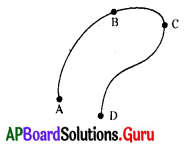A) A, B
B) A, C
C) A, D
D) B, D
C) A, D

Question 59.

 Student Time taken to reach A to B stations A 180 sec. B 230 sec. C 148 sec. D 133 sec.

Who has greatest average speed ?
A) A
B) B
C) C
D) D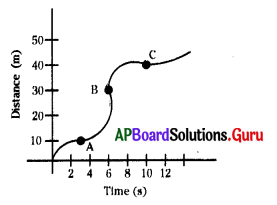D) D

Question 60.
The average speed between B to C is
A) 1.5 m/s
B) 2.5 m/s
C) 2m/s
D) 4m/s
B) 2.5 m/s

Question 61.
In the above graph speed is high at
A) A
B) B
C) C
D) speed is equal
B) B

Question 62.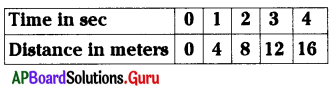The s, t graph is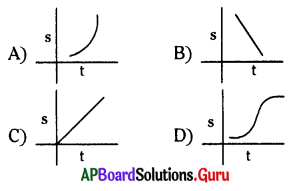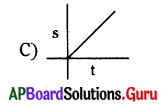Question 63.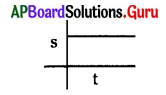The graph represents
A) uniform motion
B) non – uniform motion
C) no motion
D) constant motion
C) no motion

Question 64.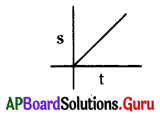The graph represents
A) uniform motion
B) non – uniform motion
C) no motion
D) circular motion
A) uniform motionQuestion 65.A particle ‘x’ travelling in uniform circular motion. Then its
A) speed is constant and velocity is also constant.
B) speed is constant and velocity changes.
C) speed changes and velocity Is con stant.
D) speed and velocity are change.
B) speed is constant and velocity changes.

Question 66.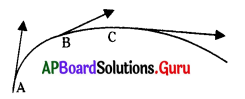From the given diagram we can say that the object.
A) has maximum speed at ‘C’
B) travelled in uniform motion.
C) has minimum speed at ‘A’.
D) A and C
D) A and C

Question 67.
v = u + at …………………. 1
s = ut + $$\frac{1}{2}$$ at2 ……………….. 2
v2 = 2as + u2 …………………. 3
v2 + u2 = 2as …………………. 4
Which of the above is not a equation of uniform acceleration ? ( )
A) 1
B) 3
C) 4
D) 1, 2, 3
C) 4

Question 68.
This graph represents the body is moving with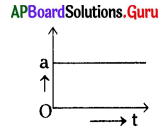A) uniform acceleration
B) uniform speed
C) uniform deceleration
D) constant speed
A) uniform acceleration

Question 69.
A particle moves along the side of a square of length ‘l’ starting from A and reaches the opposite comer ‘C’ by travelling from A to B and B to C in time the average velocity is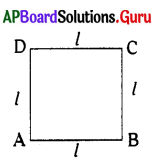A) $$\frac{2 l}{\mathrm{t}}$$
B) $$\frac{l}{\sqrt{2} \mathrm{t}}$$
C) Zero
D) $$\frac{\sqrt{2} l}{t}$$
D) $$\frac{\sqrt{2} l}{t}$$

Question 70.
Slope of the distance vs time graph
A) displacement
B) speed
C) velocity
D) acceleration
B) speed

Question 71.
Vector can be represented by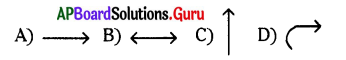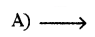Question 72.
The displacement vector to A and B points in the given figure is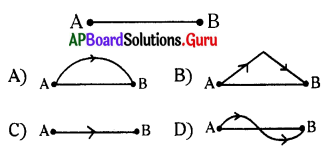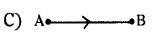Question 73.
Draw the displacement vector to AB.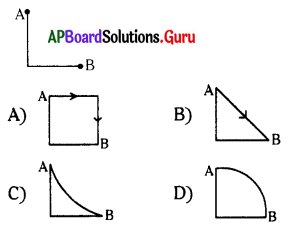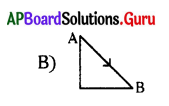Question 74.
The diagram of the direction of velocity at point ‘M’ of path when a body moves from P to Q.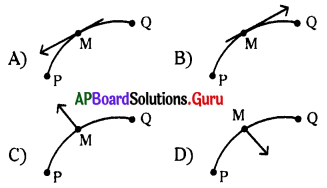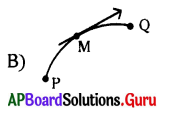Question 75.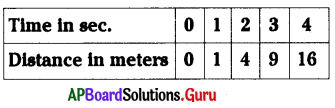If we draw the s, t graph, it look like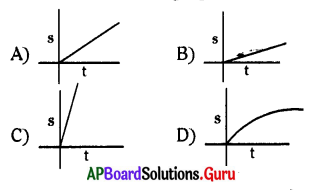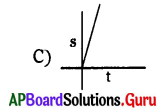Question 76.
If the average speed of a bus is 40 m/s. What is the time to travel a distance of 12 km?
A) 5 min
B) 300 min
C) 480 min
D) None
A) 5 min

Question 77.
Sridevi uses a scooter to go her office. The initial and final readings of speedometer are 4849 and 5549 respectively. The total time taken to travel that distance is 25 h. The average speed of her daily journey is
A) 28 m/hr
B) 28 km/hr
C) 2800 m/sec
D) 2.8 km/hr
B) 28 km/hrQuestion 78.
The device that shows the average speed of the vehicle is
A) Speedometer
B) Gear box
C) Odometer
D) A or C
D) A or C

Question 79.
A particle on a blade of the electrical fan moves in
A) uniform motion
B) uniform speed
C) circular path
D) above all
D) above all

Question 80.
ACB is an arc of a circle and ADB is the diameter of that circle.
The ratio of distance and displacement from A to B is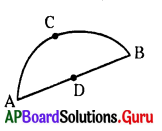A) π
B) $$\frac{\pi}{2}$$
C) 2π
D) $$\frac{\pi}{4}$$
B) $$\frac{\pi}{2}$$

Question 81.
A car was moved from A to B of distance 4800 m in 4 hrs. If its speed is 10 m/s, the ratio of distance and dis-placement between A to B is
A) 1 : 2
B) 2 : 1
C) 1: 1
D) 1 : 5
B) 2 : 1

Question 82.
Motion of a rocket: (a) ::
Motion of a satellite around the earth: (b)
A) (a) = uniform; (b) = non – uniform
B) (a) = non – uniform; (b) = uniform
C) (a) = uniform; (b) = uniform
D) (a) = non – uniform;
(b) = non – uniform
B) (a) = non – uniform; (b) = uniform

Question 83.
An apple is falling from a tree. It has
A) constant speed
B) constant velocity
C) constant direction
D) B and C
C) constant direction

Question 84.
If we are travelling in a bus, when the driver presses the acceleration, our bodies press against the seat due to
A) acceleration
B) uniform motion
C) deceleration
D) none
A) accelerationQuestion 85.
Deceleration observes in
A) moving train comes to rest
B) starting of the train
C) both A and B
D) motion of earth
A) moving train comes to rest

Question 86.
A ball is thrown upwards with 10 m/s velocity after 1 sec, what is its height?
A) 10 m
B) 5 m
C) 15 m
D) 0 m
B) 5 m

Question 87.
The speedometre of a bike gives
A) instantaneous speed
B) uniform speed
C) uniform velocity
D) acceleration
A) instantaneous speed

Question 88.
A body is moving with initial velocity 30 m/s. After certain time it acquires velocity of 40 m/s, then the velocity in middle of its journey is
A) 25√2
B) 50
C) 25
D) $$\frac{\sqrt{50}}{2}$$
A) 25√2

Question 89.
With respect to passengers in a moving bus the state of motion of tree is ………………….
A) rest
B) motion in same direction
C) motion in opposite direction
D) none of these
C) motion in opposite direction

Question 90.
When we apply brakes to car then travels with ………………………
A) acceleration
B) constant velocity
C) retardation
D) none of these
C) retardation

Question 91.
What happens if we remove mudguard from two wheelers predict ?
A) Mud moves tangentially in all directions.
B) The look of the two wheeler increases.
C) Mud will stick to the wheels and speed decreases.
D) Mud will not fall on the two wheeler.
C) Mud will stick to the wheels and speed decreases.Question 92.
Observe the figure given and the wrong question regarding relative position :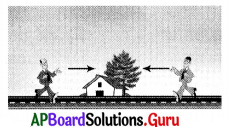A) On which side of the road is the tree located ?
B) On which side of the tree is the house located ?
C) Where is the house located with respect to road ?
D) How many persons are there in the figure ?
D) How many persons are there in the figure ?

Question 93.
The experimental arrangement correct order is:
a. Keep the steel plate on the floor at the bottom of the tube.
b. Mark the readings in cm along the tube.
c. Take a long plastic tube of length nearly 200 cm which is be into half along the length.
d. Place one end of the tube on the book and the other end on the floor.
A) c, d, b, a
B) c, d, a, b
C) c, b, d, a
D) a, b, c, d
B) c, d, a, b

Question 94.
The apparatus which is missing in the above question (3) to perform the experiment is
a. Glass marble
b. Digital stop clock
c. Thermocol ball
A) a and b
B) c only
C) b only
D) a, b and c
A) a and b

Observe the table given below answer the questions 5 and 6: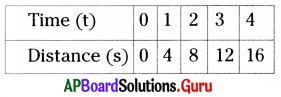Question 95.
What do you generalise from the table given above ?
A) Non-uniform velocity
B) Rest
C) Accelerated motion
D) Uniform velocity
D) Uniform velocity

Question 96.
The correct graph representing the above table is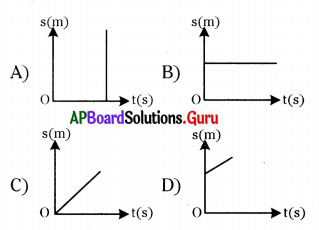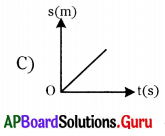Question 97.
The distance(s) – time(t) graph which represents rainy drops which are falling from certain height with uniform velocity is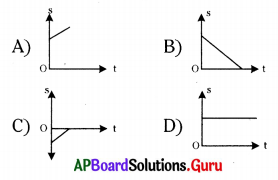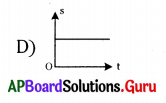Question 98.
A car covers half the distance at a speed of 60 kmh-1 and the other half at a speed of 40 km h-1. The average speed of the car is
A) 44.44 km h-1
B) 50 km h-1
C) 48 km h-1
D) 0
B) 50 km h-1

Question 99.
Mithilesh wanted to find instantaneous speed of the car in which he was travelling. He should see which one of the following meters ?
A) Anaemometer
B) Odometer
C) Speedometer
D) Thermometer
C) Speedometer

Question 100.
An example for a motion where the speed and direction of motion both changes continuously is
A) Ball moving down the inclined plane
B) Throwing stone into the air, making some angle with the horizontal
C) Ball moving up the inclined plane
D) Whirling stone
B) Throwing stone into the air, making some angle with the horizontal

Question 101.
Velocity : ms-1 :: acceleration :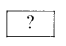A) ms-2
B) m2s
C) m-2s
D) ms2
A) ms-2

Question 102.
When the driver pushes the accelerator of the car, then
A) the time interval to reach the destination decreases.
B) the time interval to reach the destination remains the same.
C) the speed and time interval both increase.
D) the time interval to reach the destination increases.
A) the time interval to reach the destination decreases.

Question 103.
In the below graph, the velocity in ms-1 is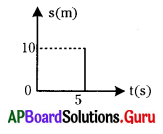A) 1/2 ms-1
B) Not defined
C) 50 ms-1
D) 2 ms-1
D) 2 ms-1

Question 104.
Which of the following is not a vector?
A) Speed
B) Acceleration
C) Velocity
D) Displacement
A) SpeedQuestion 105.
The velocity of the car was measured by a traffic police through his radar gun at that instant. The gun measured
A) Instantaneous acceleration
B) Instantaneous velocity
C) Average acceleration
D) Average velocity
B) Instantaneous velocity

Question 106.
Siva completed half revolution of circular path of radius ‘a’ units. Displace-ment of Siva is
A) ‘a’ units
B) ‘2a’ units
C) πa units
D) 2πa units
B) ‘2a’ units

Question 107.
Acceleration of a person moving with constant velocity
A) Infinite
B) Positive acceleration
C) Negative acceleration
D) Zero
D) Zero

Question 108.
Thanish starts at 8 am to Ananthapuram from Amaravathi and reaches at 6pm. The distance between Amaravathi and Ananthapuram is 500 km. Average velocity of Thanish is
A) 0 km / hour
B) 40 km / hour
C) 50 km / hour
D) 60 km / hour
C) 50 km / hour

Question 109.
A particle is moving in a circular path of radius r. What would be the displacement after completely distance of exactly one circle i.e ‘r’ ?
A) 0
B) r
C) 2r
D) $$\frac{r}{2}$$
A) 0

Question 110.
Which of the following is a scalar quantity ?
A) Velocity
B) Momentum
C) Impulse
D) Mass
D) Mass

Question 111.
What does the slope of a displacement and time graph represent ?
A) Mass
B) Velocity
C) Acceleration
D) Momentum
B) VelocityQuestion 112.
The ratio of speed and velocity is
A) Always equal to 1
B) Always greater than 1
C) Always less than 1
D) Depends on the direction of motion
B) Always greater than 1

Question 113.
Based on the direction find the Odd one.
A) Velocity
B) Displacement
C) Speed
D) Acceleration
C) Speed

Question 114
……………….. is speed in Specified direction.
A) Distance
B) Velocity
C) Acceleration
D) Displacement
B) Velocity

Question 115.
Match the following equations of uniform accelerated motion formulae: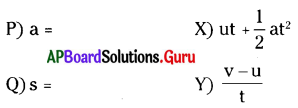A) P -X, Q – Y, R – Z
B) P – Y, Q – X, R – Z
C) P – Z, Q – X, R – Y
D) P – Y, Q – Z, R – X
B) P – Y, Q – X, R – Z

Question 116.
Distance – time graph is shown in the figure.
Predict the equivalent velocity – time graph
among the following is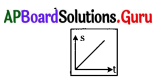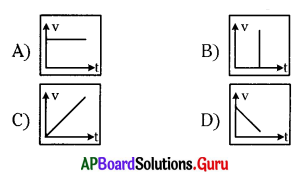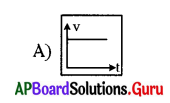Question 117.
Which of the following is Non-Uniform motion :
A) Motion of a ball on inclined plane
B) Uniform circular motion
C) A stone thrown in air
D) All the above
D) All the aboveQuestion 118.
If the equation of motion of an object is v2 = 2as, the initial velocity is ……….
A) Zero
B) Infinity
C) 10 m/s
D) We can’t say
A) Zero

Question 119.
Which of the following graph indicate uniform velocity ?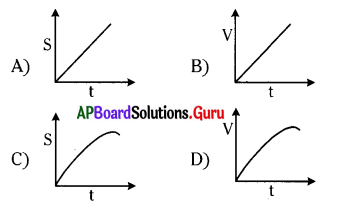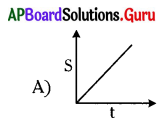Question 120.
For a vector A B which of the following is correct ?
A) length indicates magnitude
B) arrow indicates direction
C) both A and B
D) is a scalar